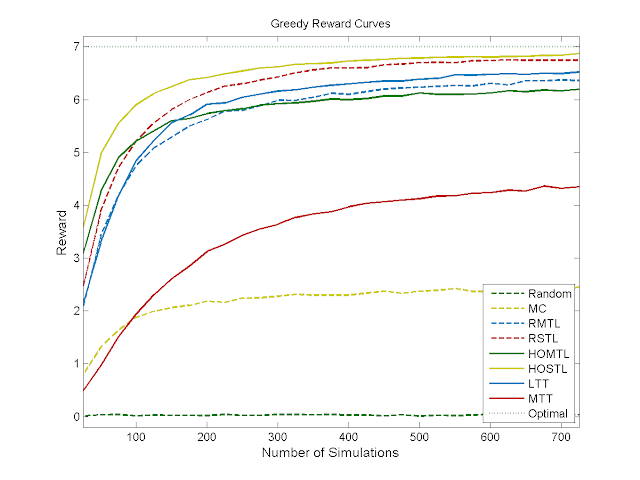Monday, June 4, 2012

Feedback 04-06-2012

Results
Donut World

1. Step limit: 10 steps per episode
2. Reward: +1 for being on the donut, 0 otherwise
3. Gaussian noise added to the rewards: mean = 0, sigma = 0.5
4. Optimal reward equals 7 because of turning the first 3 steps
5. Results averaged over 10,000 episodes
6. Algorithm MC randomly samples 10-step trajectories and plays the best found
7. LTT is the "Level-based Transposition Tree" with first a regression tree discretizing the state space and each leaf of this tree holds a regression tree discretizing the action space (for that region of the state space).
8. MTT is the "Mixed Transposition Tree" which is only one regression tree but also splits on the state space.9. Random performs worst, followed by MC.
10. Performance wise, STL > RTL and then HOO > IRTI
11. LTT performs about average in comparison with the four algorithms mentioned above. MTT performs bad in comparison with the other algorithms (probably due to the problem stated in the previous post), but is still better than MC and Random.12. Obviously MC and Random are the fastest algorithms
13. HOO is the slowest and the plot shows its running time of O(n^2)
14. TT is a bit slower than RMTL and RSTL, probably due to that fact that they re-use the same tree build in previous real-world steps.
15. Initially MTL is a bit faster than STL, but MTL's computational complexity is slightly higher (due to the perfect recall).